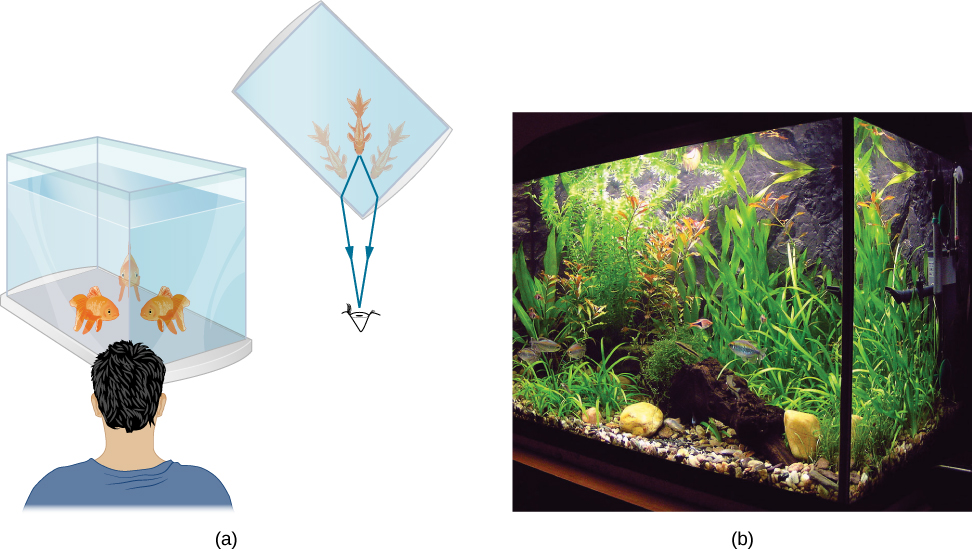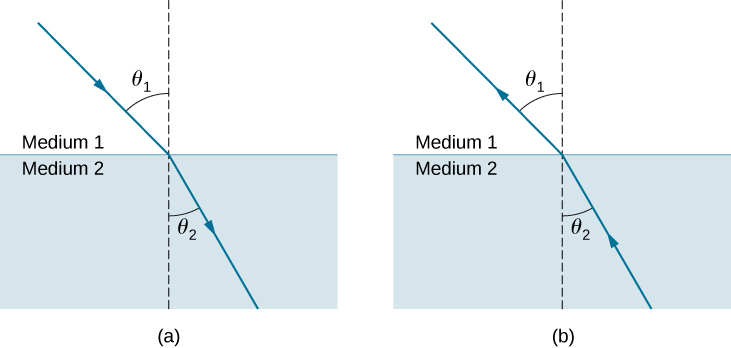# 1.3 Refraction

 Page 1 / 4
By the end of this section, you will be able to:
• Describe how rays change direction upon entering a medium
• Apply the law of refraction in problem solving

You may often notice some odd things when looking into a fish tank. For example, you may see the same fish appearing to be in two different places ( [link] ). This happens because light coming from the fish to you changes direction when it leaves the tank, and in this case, it can travel two different paths to get to your eyes. The changing of a light ray’s direction (loosely called bending) when it passes through substances of different refractive indices is called refraction    and is related to changes in the speed of light, $v=c\text{/}n$ . Refraction is responsible for a tremendous range of optical phenomena, from the action of lenses to data transmission through optical fibers.(a) Looking at the fish tank as shown, we can see the same fish in two different locations, because light changes directions when it passes from water to air. In this case, the light can reach the observer by two different paths, so the fish seems to be in two different places. This bending of light is called refraction and is responsible for many optical phenomena. (b) This image shows refraction of light from a fish near the top of a fish tank.

[link] shows how a ray of light changes direction when it passes from one medium to another. As before, the angles are measured relative to a perpendicular to the surface at the point where the light ray crosses it. (Some of the incident light is reflected from the surface, but for now we concentrate on the light that is transmitted.) The change in direction of the light ray depends on the relative values of the indices of refraction ( The Propagation of Light ) of the two media involved. In the situations shown, medium 2 has a greater index of refraction than medium 1. Note that as shown in [link] (a), the direction of the ray moves closer to the perpendicular when it progresses from a medium with a lower index of refraction to one with a higher index of refraction. Conversely, as shown in [link] (b), the direction of the ray moves away from the perpendicular when it progresses from a medium with a higher index of refraction to one with a lower index of refraction. The path is exactly reversible.The change in direction of a light ray depends on how the index of refraction changes when it crosses from one medium to another. In the situations shown here, the index of refraction is greater in medium 2 than in medium 1. (a) A ray of light moves closer to the perpendicular when entering a medium with a higher index of refraction. (b) A ray of light moves away from the perpendicular when entering a medium with a lower index of refraction.

The amount that a light ray changes its direction depends both on the incident angle and the amount that the speed changes. For a ray at a given incident angle, a large change in speed causes a large change in direction and thus a large change in angle. The exact mathematical relationship is the law of refraction    , or Snell’s law , after the Dutch mathematician Willebrord Snell (1591–1626), who discovered it in 1621. The law of refraction is stated in equation form as

A Pb wire wound in a tight solenoid of diameter of 4.0 mm is cooled to a temperature of 5.0 K. The wire is connected in series with a 50-Ωresistor and a variable source of emf. As the emf is increased, what value does it have when the superconductivity of the wire is destroyed?
how does colour appear in thin films
in the wave equation y=Asin(kx-wt+¢) what does k and w stand for.
derivation of lateral shieft
hi
Imran
total binding energy of ionic crystal at equilibrium is
How does, ray of light coming form focus, behaves in concave mirror after refraction?
Sushant
What is motion
Anything which changes itself with respect to time or surrounding
Sushant
good
Chemist
and what's time? is time everywhere same
Chemist
No
Sushant
how can u say that
Chemist
do u know about black hole
Chemist
Not so more
Sushant
DHEERAJ
Sushant
But ask anything changes itself with respect to time or surrounding A Not any harmful radiation
DHEERAJ
explain cavendish experiment to determine the value of gravitational concept.
Cavendish Experiment to Measure Gravitational Constant. ... This experiment used a torsion balance device to attract lead balls together, measuring the torque on a wire and equating it to the gravitational force between the balls. Then by a complex derivation, the value of G was determined.
Triio
For the question about the scuba instructor's head above the pool, how did you arrive at this answer? What is the process?
as a free falling object increases speed what is happening to the acceleration
of course g is constant
Alwielland
acceleration also inc
Usman
which paper will be subjective and which one objective
jay
normal distributiin of errors report
Dennis
normal distribution of errors
Dennis
acceleration also increases
Jay
there are two correct answers depending on whether air resistance is considered. none of those answers have acceleration increasing.
Michael
Acceleration is the change in velocity over time, hence it's the derivative of the velocity with respect to time. So this case would depend on the velocity. More specifically the change in velocity in the system.
Big
photo electrons doesn't emmit when electrons are free to move on surface of metal why?
What would be the minimum work function of a metal have to be for visible light(400-700)nm to ejected photoelectrons?
give any fix value to wave length
Rafi
40 cm into change mm
40cm=40.0×10^-2m =400.0×10^-3m =400mm. that cap(^) I have used above is to the power.
Prema
i.e. 10to the power -2 in the first line and 10 to the power -3 in the the second line.
Prema
there is mistake in my first msg correction is 40cm=40.0×10^-2m =400.0×10^-3m =400mm. sorry for the mistake friends.
Prema
40cm=40.0×10^-2m =400.0×10^-3m =400mm.
Prema
this msg is out of mistake. sorry friends​.
Prema
what is physics?
why we have physics
because is the study of mater and natural world
John
because physics is nature. it explains the laws of nature. some laws already discovered. some laws yet to be discovered.
Yoblaze
physics is the study of non living things if we added it with biology it becomes biophysics and bio is the study of living things tell me please what is this?
tahreem
physics is the study of matter,energy and their interactions
Buvanes
all living things are matter
Buvanes
why rolling friction is less than sliding friction
tahreem
thanks buvanas
tahreem